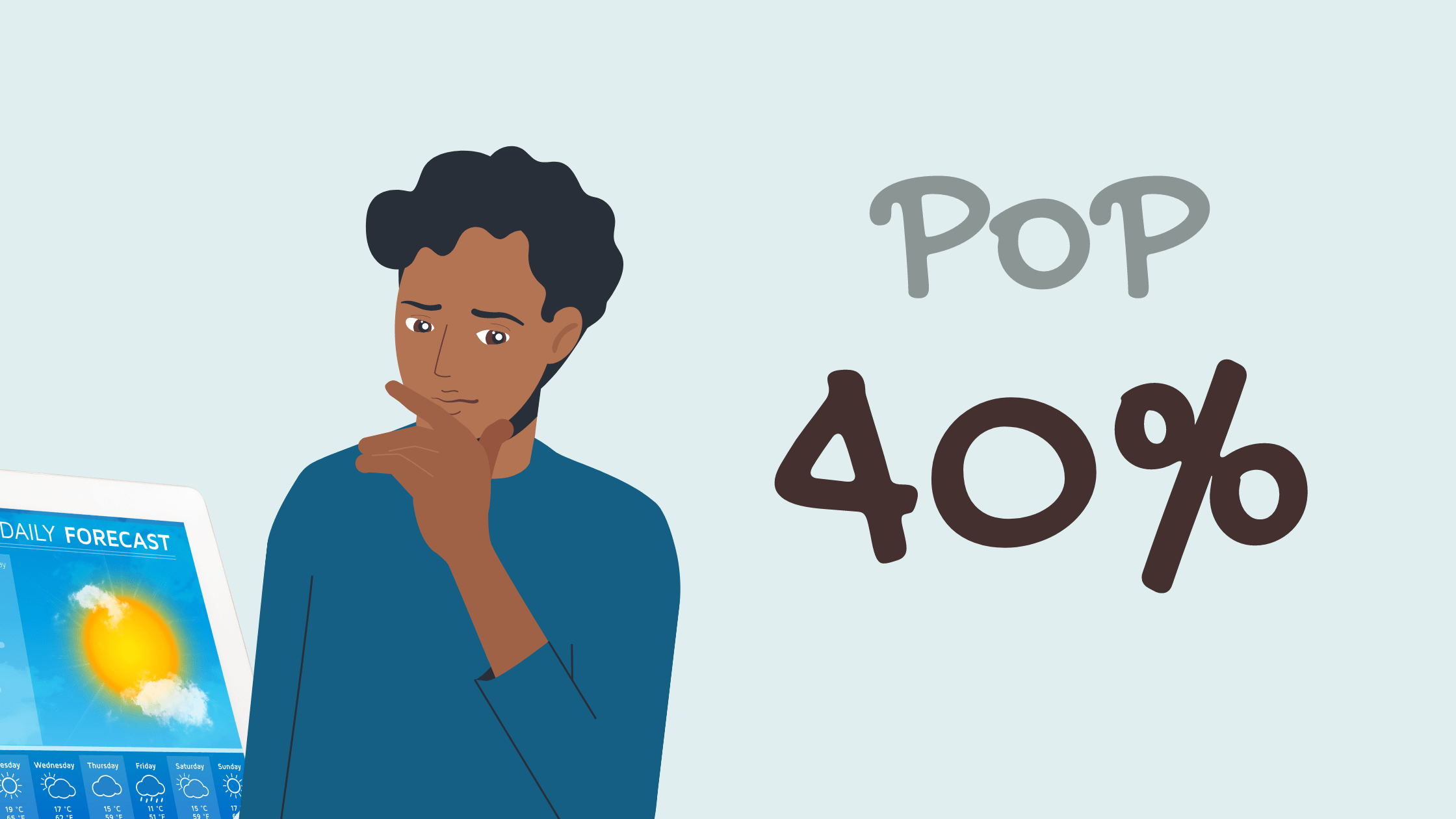# What Does the Percentage of Rain Mean?Whenever we check the weather forecast and they call for rain, we see the percentage of rain. But, what does the percentage of rain mean? Is it the likelihood of it raining? Or is it how much of the area is going to be covered by the rain?

Percentages are used to describe and represent different things. They can be used to determine the probability of an event occurring or show a portion of a whole for any quantity.

One whole given as a percent is 100%.

When we are stating a fraction of a whole, we can express it as a decimal and as a percent: 1/100  = 0.01 = 1%; ¼ = 0.25 = 25%; ½ = 0.5 = 50% and so on.

When we see the percentage of rain in the weather forecast, it often comes with the abbreviation “PoP”.

“PoP” stands for “Probability of Precipitation”, where “precipitation” is the rain.

Thus, the abbreviation itself partially reveals the meaning of the number in % – it is supposed to mean the probability of rain occurring.

However, recently there has been some confusion as to whether that is the case.

So, let’s confirm.

According to the National Weather Service (USA) “PoP 40%, 0.01” means that there is a 40% probability that the forecast point in question will have at least one hundredth of an inch of rain.

Environment Canada uses the term “Chance of Precipitation” (CoP) that is defined as “the chance that measurable precipitation (0.2 mm of rain or 0.2 cm of snow) will fall on any random point of the forecast region during the forecast period.” The values are given in 10% increments. The use of 50% is not permitted.

Based on the above, it is clear that the answer to the question “what does the percentage of rain mean?” is that it means the probability of a certain amount of rainfall in the forecast region (however, not necessarily evenly distributed).

Now, is PoP of 40% high or low?

Probability is measured with the help of a probability line from 0 to 1 (or 0% to 100%) with a half-mark in the middle – 0.5 (50%).

Anything to the right of 50% is likely all the way to certain. Anything to the left of 50% is unlikely all the way to impossible. 50% means equally likely.

If the PoP is given as 40%, it falls under the “unlikely” category, however, definitely not impossible. This could mean a short period(s) of sporadic rain.